The paper “Neural Machine Translation By Jointly Learning To Align And Translate” introduced in 2015 is one of the most famous deep learning paper related natural language process which is cited more than 2,000 times. This article is a quick summary of the paper.

# Introduction & Background

## Machine Translation (MT)

Machine translation is a sub-field of computational linguistics that investigates the use of software to translate text or speech from one language to another. - Wikipedia

• Neural MT
• Train a large neural network, read a sentence and output a correct translation (i.e. RNN encoder-decoder model).
• In probabilistic perspective, translation is equivalent to find a target sentence $y$ that maximizes the conditional probability of $y$ given a source sentence $x$ (i.e. $argmax_y P(y \mid x)$).
• In neural MT, we fit a parameterized model to maximize the conditional probability of sentence pairs using a parallel training corpus.
• Once the conditional distribution is learned by a translation model, given a source sentence a corresponding translation can be generated by searching for the sentence that maximizes the conditional probability.

## RNN Encoder - Decoder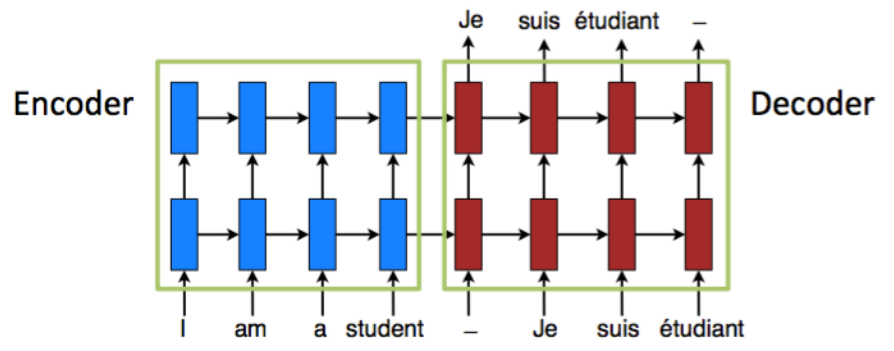• RNN Encoder - Decoder model is previous work of the author on the paper “Learning Phrase Representations using RNN Encoder–Decoder for Statistical Machine Translation” in 2014.
• RNN Encoder - Decoder model is usually known as a sequence-to-sequence (seq2seq) model and it has advantage to understand the sentence correctly since the whole context of the sentence should be considered through context vector $c$ that is encoded from input sentence.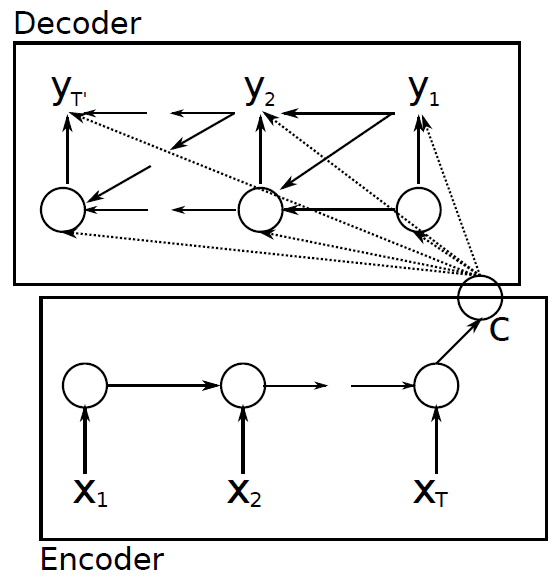• Encoder
• Reads the input sentences, a sequence of vectors $x=(x_1, ..., x_{T_x})$, into a vector $c$.
• Used LSTM model.
• Decoder
• Predict the next word $y_t$ given the context vector $c$.
• Defines a probability over the translation $y$ by decomposing the joint probability into the ordered conditional probabilities.
• Each conditional probability is modeled by the function $g$, which is nonlinear, potentially multi-layered, outputs the probability of $y_t$ when $s_t$ is a hidden state of the RNN.
• Used LSTM model.
• Problem of RNN Encoder - Decoder model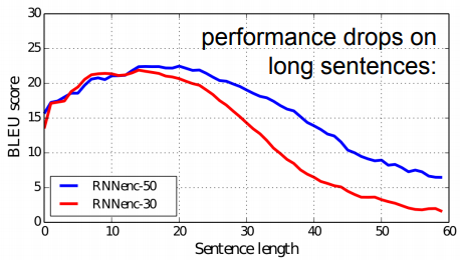• Potential issue is that the network needs to be able to compress all the necessary information of the source sentence into a fixed-length vector. It is difficult for the neural network to process a long sentences, especially those that are longer than the sentences in the training corpus.
• Decoder uses only one context vector $c$ to generate output words in all of the RNN steps. However, each output world has to be influenced by different input words respectively.

# Learning to Align and Translate

## Encoder: Bidirectional RNN for Annotating Sequences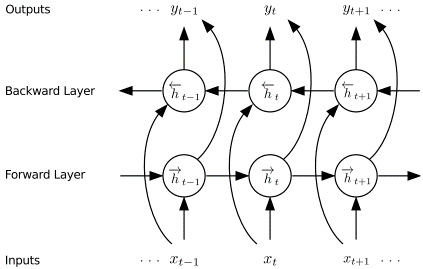• Use bidirectional RNN for annotating sequences which consists of forward and backward RNN.
• This summarize not only the preceding words but also the following words
• Backward RNN $\overleftarrow{f}$
• Reads the input sequence in reverse order $(x_{T_x}, ..., x_1)$
• Hidden states: $(\overleftarrow{h_1}, ..., \overleftarrow{h_{T_s}})$
• Forward RNN $\overrightarrow{f}$
• Reads the input sequence in proper order $(x_1, ..., x_{T_x})$
• Hidden states: $(\overrightarrow{h_1}, ..., \overrightarrow{h_{T_x}})$
• $(h_1, ..., h_L)$ is the new variable-length representation instead of fixed-length $c$.
• Annotation for each word $h_j$: $h_j = [\overrightarrow{h_{j}^{T}};\overleftarrow{h_{j}^{T}}]^{T}$
• $h_j$ contains $x_j$ together with its context $(..., x_{j-1}, x_{j+1}, ...)$.

## Decoder: General Description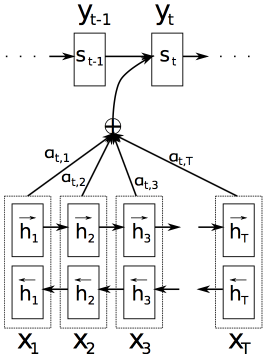• Define each conditional probability: $p(y_i \mid y_1, ..., y_{i-1}, x) = g(y_{i-1}, s_i, c_i)$
• $s_i$ is an RNN hidden state for time $i$: $s_i = f(s_{i-1}, y_{i-1}, c_i)$
• Context $c_i$ is computed as a weighted sum of $h$:
• Alignment model $(a)$ parameterizes it as a feedforward neural network which is jointly trained with all the other components of the system.

# Experiment and Result

## Dataset

• Evaluate the proposed approach on the task of English-to-French translation
• Use ACL WMT’14 dataset: bilingual, parallel corpora
• Europarl (61M words), News commentary (5.5M), UN (421M), Two crawled corpora of 90M and 272.5M words, total 850M words
• Reduce the size of the combined corpus to have 348M
• Concatenate news-test-2012 and news-test-2013 to make a validation set, and evaluate the models on the test set (news-test-2014)

## Models

• Train two types of models
• RNN Encoder-Decoder (refer as RNNencdec): Baseline, 1000 hidden units
• RNNsearch: Proposed model, 1000 hidden units
• To train the models,
• Use a multiplayer network with a single maxout hidden layer
• Use a mini-batch stochastic gradient descent (SGD)
• Use a beam search to find a translation that approximately maximizes the conditional probability

## Quantitative Results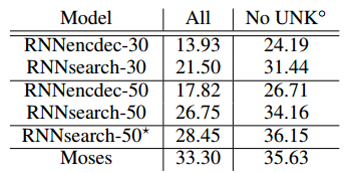• The translation performance measured in BLEU score
• RNNsearch outperforms the RNNencdec for all the cases
• RNNsearch is as high as that of the conventional phrase-based translation system (Moses)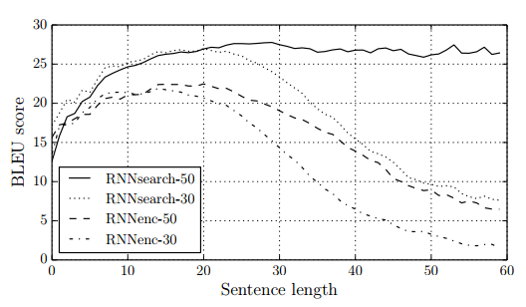• The performance of RNNencdec dramatically drops as the length of the sentences increases
• Both RNNsearch-30 and RNNsearch-50 are more robust to the length of the sentences
• Especially, RNNsearch-50 shows no performance deterioration even with sentences of length 50 or more

## Quantitative Analysis

### Alignment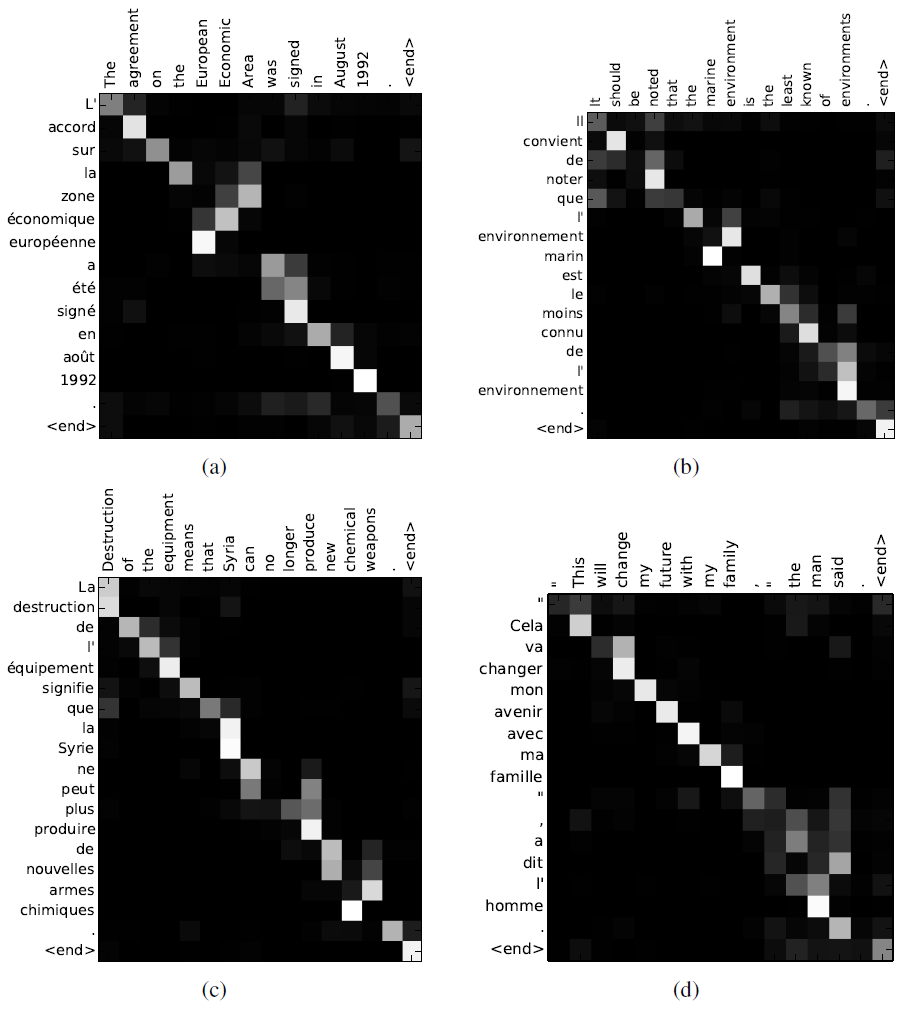• Four sample alignments found by RNNsearch-50 between the source sentence (English) and the generated translation (French)
• Each pixel shows the weight $\alpha_ij$ of the annotation of the $j$-th source word for the $i$-th target word, in grayscale (0:black, 1:white)
• (a): an arbitrary sentence, (b-d): three randomly selected samples among the sentences without any unknown words and of length between 10 and 20 words from the test set

### Long Sentences

• Source sentence from the test set:

An admitting privilege is the right of a doctor to admit a patient to a hospital or a medical centre to carry out a diagnosis or a procedure, based on his status as a health care worker at a hospital.

• The RNNencdec-50 translated this sentence into:

Un privilege d’admission est le droit d’un m´edecin de reconnaˆıtre un patient al’hˆopital ou un centre m´edical d’un diagnostic ou de prendre un diagnostic enfonction de son ´etat de sant´e.

• It correctly translated the the source sentence until [a medical center], but it deviated afterward
• The RNNsearch-50 generated the following correct translation:

Un privilege d’admission est le droit d’un m´edecin d’admettre un patient a unhˆopital ou un centre m´edical pour effectuer un diagnostic ou une proc´edure, selonson statut de travailleur des soins de sant´e `a l’hˆopital.

• It preserve the whole meaning of the input sentence without omitting any details
• This is because the RNNsearch does not require encoding a long sentence into a fixed-length vector, but only accurately encoding the parts of the input sentence that surround a particular word.

# Conclusion

• The conventional approach (encoder-decoder) issues
• Encodes a whole input sentence into a fixed-length vector
• it cannot translate long sentences
• Propose a novel approach by extending the basic encoder-decoder
• No fixed size representation
• Plausible alignment
• Neural Machine Translation by Jointly Learning to Align and Translate [Link]
• Learning Phrase Representations using RNN Encoder-Decoder for Statistical Machine Translation [Link]